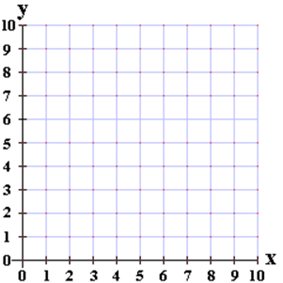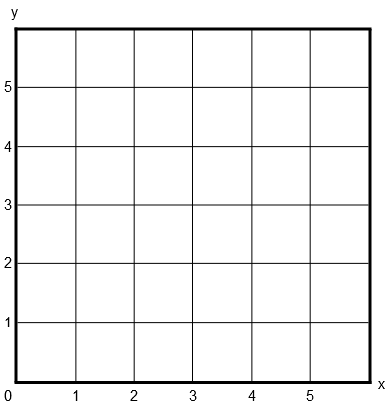# Patterns and the Coordinate Plane

## Objective

Construct a coordinate plane and plot points given their coordinates.

## Common Core Standards

### Core Standards

?

• 5.G.A.1 — Use a pair of perpendicular number lines, called axes, to define a coordinate system, with the intersection of the lines (the origin) arranged to coincide with the 0 on each line and a given point in the plane located by using an ordered pair of numbers, called its coordinates. Understand that the first number indicates how far to travel from the origin in the direction of one axis, and the second number indicates how far to travel in the direction of the second axis, with the convention that the names of the two axes and the coordinates correspond (e.g., x-axis and x-coordinate, y-axis and y-coordinate).

?

• 2.MD.B.6

## Criteria for Success

?

1. Given the coordinates of a point, plot it on a coordinate plane with intervals of 1 unit.
2. Understand that there are two different ways of interpreting a point, e.g., (2, 3): (1) as instructions (right 2, up 3) and (2) as the point defined by being a distance 2 from the y-axis and a distance 3 from the x-axis.
3. Given instructions from another point on a coordinate plane, plot a point on a coordinate plane with intervals of 1 unit.

## Tips for Teachers

?

#### Remote Learning Guidance

If you need to adapt or shorten this lesson for remote learning, we suggest prioritizing Anchor Task 2 (benefits from worked example). Find more guidance on adapting our math curriculum for remote learning here.

#### Fishtank Plus

• Problem Set
• Student Handout Editor
• Vocabulary Package

?

### Problem 1

Part A:

Plot a point on the coordinate grid below at (2, 6).Part B:

Alex says that the ordered pair (2, 6) is 2 units from the x-axis and 6 units from the y-axis. Do you agree or disagree?

#### References

Grade 5, Unit 9, Lesson 2 (2017-2018) is made available by Achievement First as a part of their Open Source web portal under a CC BY 4.0 license. Copyright © 1999-2017 Achievement First. Accessed May 25, 2018, 3:09 p.m..

Modified by The Match Foundation, Inc.

### Problem 2

1. Plot the following points on the coordinate grid below:
• Point A: (3, 8)
• Point B: (7, 2)
• Point C: (6, 0)
• Point D: (0, 4)
• Point E: 3 units to the left and 4 units up from point B
• Point F: 5 units to the right and 2 units down from point D1. What are the coordinates of point E? Of point F

## Problem Set & Homework

#### Discussion of Problem Set

• Look at #2(b). Which shape is 2 units from the y-axis? Does this correspond with the x-coordinate or the y-coordinate?
• If point A lies at a distance of 3 units from the x-axis, which coordinate do you know?
• Where did Amy stop in #5? If someone chose point A, what error might they have made? What about point C? Point D?

?

Coordinates for points are shown in the table.

 Point Coordinate A (2, 5) B (1, 4) C (0, 3) D (1, 0)

Graph all the points from the table in the coordinate grid. Label them with their corresponding letters.#### References

PARCC Released Items Math Spring 2017 Grade 5 Released ItemsQuestion #4

### Mastery Response

?